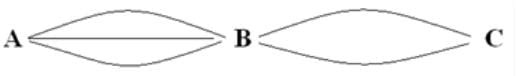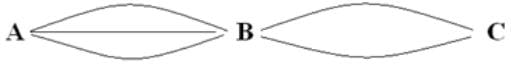# Permutation & Combination Notes | Study CSAT Preparation for UPSC CSE - UPSC

## UPSC: Permutation & Combination Notes | Study CSAT Preparation for UPSC CSE - UPSC

The document Permutation & Combination Notes | Study CSAT Preparation for UPSC CSE - UPSC is a part of the UPSC Course CSAT Preparation for UPSC CSE.
All you need of UPSC at this link: UPSC

What is Permutation?

Permutation relates to the act of arranging all the members of a set into some sequence or order.
• In other words, if the set is already ordered, then the rearranging of its elements is called the process of permuting.
• Permutations occur, in more or less prominent ways, in almost every area of mathematics. They often arise when different orderings on certain finite sets are considered.

What is a Combination?

The combination is a way of selecting items from a collection, such that (unlike permutations) the order of selection does not matter.
• In smaller cases, it is possible to count the number of combinations. Combination refers to the combination of n things taken k at a time without repetition.
• To refer to combinations in which repetition is allowed, the terms k-selection or k-combination with repetition are often used.

The Fundamental Principle of Counting

Product Rule

If an activity A can be done in “m” ways and another activity “B” can be done in “n” ways, A and B together can be done in “m x n” ways.

Example 1: How many ways a person can go from A to C via B?A to B in 3 ways

B to C in 2 ways

A to B and B to C in 3 x 2 = 6 ways

If an activity A can be done in “m” ways and another activity “B” can be done in “n” ways, then A or B together can be done in “m + n” ways.
Example 2: If the person is at B, how many ways he can go to A or C?B to A in 3 ways
B to C in 2 ways
B to A OR B to C in 3 + 2 = 5 ways

Keywords
AND➜ MULTIPLICATION (Product rule)

Q.1. From a pack of cards in how many ways can you select-

a) A king and a queen

b) A king or a queen

There are 52 cards in a pack, with 4 kings and 4 queens.

A king can be selected in 4 ways and a queen can be selected in 4 ways. Number of ways of selection of a king AND a queen is 4×4=16 (Product rule)

Number of ways of selection of a king OR a queen 4+4=8 (Addition rule)

The fundamental principle of counting (FPC) is the basic concept of permutation and combination.

Q.2. In how many ways can 3 people A, B, C be arranged in 2 places?

Manually solving we will get 6 cases as given below.

AB BA

AC CA

BC CB

This can be explained using FPC like this

There are two seats __ __. In the first seat A,B or C can sit. i.e., 3 possibilities.

Let A sit in the first place. Thus, in second place either B or C can sit.

The second seat can hence be taken up in 2 ways Together A,B ‘AND’ C can be seated in 3 x 2 = 6 ways.

Thus, the number of arrangement of 3 things at 2 places can be done in 3×2 ways.

Q.3. In how many ways can 4 people be seated in 3 chairs?

First seat can be filled in 4 ways.

Second seat can be filled in 3 ways.

Third seat can be filled in 2 ways.

So all together 4 people can be arranged in 3 seats in 4x3x2 = 24 ways.

Generalizing; arrangement of n things at r places can be done in [n(n-1)(n-2)…(n-r+1)] ways.

This arrangement of n things at r places is denoted by nPr .

nPr = n(n-1)(n-2)…(n-r+1) = n!/(n-r)!

It should be noted that in all these examples, the order of arrangement is important.

In other words, AB and BA are counted as 2 different cases. An arrangement where the order is important is called permutation

Now let us see one case where the order is not important.

Try yourself:In how many different ways can the letters of the word 'LEADING' be arranged in such a way that the vowels always come together?

Q.4. How many doubles teams can be selected from 3 persons A, B, C?

Let’s take a doubles team AB. Change the order, i.e. to BA. Notice that both the teams are one and the same.

So here the order is not important. Hence, it’s a case of a combination. Here one can select the team in 3 ways

AB

AC

BC

If the order is important, then the total number of arrangements can be done in nPr = 3P2 ways.

We will get the 6 cases as:

AB BA

AC CA

BC CB

But in this particular question every 2! (because of A,B can be arranged in 2! Ways) cases will be counted as only one

case, i.e AB and BA is one and the same team.

So the number of selections of 2 things from 3 things can be done in = (3P2) / 2!

Similarly, the number of selections of 3 things from 4 things can be done in = (4P3) / 3!

Generalizing: the number of selections of r things from n things can be done in = (nPr) / r! ways.

The number of selections of r things from n different things is denoted by nCr,

which is what we learn as “Combination”.

∴ nCr = nPr/r!=n!/[(n-r)! r!]

Thus, you can understand now, that wherever the arrangement is necessary apart from selection,

we used Permutation and when we just need to select without arranging, we use Combination.

To make the point even clearer.

Try yourself:Find the number of words, with or without meaning, that can be formed with the letters of the word ‘CHAIR’.

Q.5. In how many ways can you select a President and a Vice-President from 3 people?

Is it a case of permutation or combination?

In the above question, since the word “select” appears, it may tempt you to consider combination.

So let’s take a case where A is the President and B is the Vice-President. Now change the order,

i.e. B is President and A Vice-President. Are both the cases same? No, they are not! We see that both the cases are different.

The order is important. Hence it is a case of permutation.

Difference between Permutation and Combination:
 Permutation Combination nPr = n!/(n-r)! nCr = n!/[(n-r)!r!] Number of ways of arranging n things taken r at a time. Number of ways of selecting r things out of n things. Order is important Order is not important

The relation between permutation and combination

nPr = nCr x r!

Try yourself:Find the number of words, with or without meaning, that can be formed with the letters of the word ‘INDIA’.

The permutation of ‘n’ things taken ‘r’ at a time will involve the following steps:

(i) Select ‘r’ things out of total ‘n’ things, which can be done in nCr ways.

(ii) Arrange those ‘r’ things among themselves which can be done in r! ways.

nPr is nothing but, selecting r things out of n things (in nCr ways) and then arranging it among themselves.

This is why every permutation is selection followed by the arrangement of the selected things among themselves.

If it is only selection where the arrangement is not important, then we use the combination (nCr) only.

Try yourself:Among a set of 5 black balls and 3 red balls, how many selections of 5 balls can be made such that at least 3 of them are black balls.

Try yourself:Solve the following.
i) 30 P
ii) 30 C 2

The document Permutation & Combination Notes | Study CSAT Preparation for UPSC CSE - UPSC is a part of the UPSC Course CSAT Preparation for UPSC CSE.
All you need of UPSC at this link: UPSCUse Code STAYHOME200 and get INR 200 additional OFF

## CSAT Preparation for UPSC CSE

72 videos|64 docs|92 tests

### How to Prepare for UPSC

Read our guide to prepare for UPSC which is created by Toppers & the best Teachers

Track your progress, build streaks, highlight & save important lessons and more!

,

,

,

,

,

,

,

,

,

,

,

,

,

,

,

,

,

,

,

,

,

;